Disclosure: This post may contain affiliate links, meaning when you click the links and make a purchase, we receive a commission.

# How to calculate IRR (internal rate of return) in Excel (9 easy ways)

In this article, I will discuss how to calculate IRR (internal rate of return) in Excel. I will focus on several topics like:

• Calculating IRR using Excel’s IRR & XIRR function
• Calculating IRR using Excel’s Goal Seek feature
• Calculating IRR manually or by hand
• How to calculate IRR from NPV (net present value)
• Using IRR function in Excel VBA
• How to calculate IRR in Excel for monthly cash flow
• Calculating IRR for Uneven cash flow
• How to calculate MIRR in Excel (modified internal rate of return)
• And what is a good IRR

I hope you will enjoy the whole article.

Sam is your friend. Comes to you with an investment proposal. You are proposed to invest \$1000 and these are the cash flows you can expect for the investment over the next 5 years.

 Year Cashflow (\$) 0 -1000 (your investment) 1 200 2 500 3 200 4 150 5 500

There is a bank nearby your place and you trust this bank. And this bank provides 12% yearly interest on a deposit. Or you can invest your money in government saving bond that provides 10% interest rate/year. The government bond is more secure than depositing with a bank.

So, you have three options to invest your \$1000:

• You can invest in your friend Sam’s project
• You can deposit the amount in your preferred bank that provides 12% interest rate per year
• Or you can buy the government bond and enjoy more secured income

What will you do? To make a better decision, you have to know, at first, the internal rate of return on your investment with Sam’s project.

Let’s calculate the IRR of these cash flows. There are several scenarios of cash flows (it can be regular, discrete, monthly, etc.), so your approach will also be different when you will calculate IRR.

## 1) Calculating IRR (internal rate of return) using Excel’s IRR function (Cash Flow is Continuous/Regular)

For our above example, cash flow is continuous. So, we can easily calculate IRR using Excel’s IRR function.

In cell K11, I have placed this formula: =IRR(K4:K9)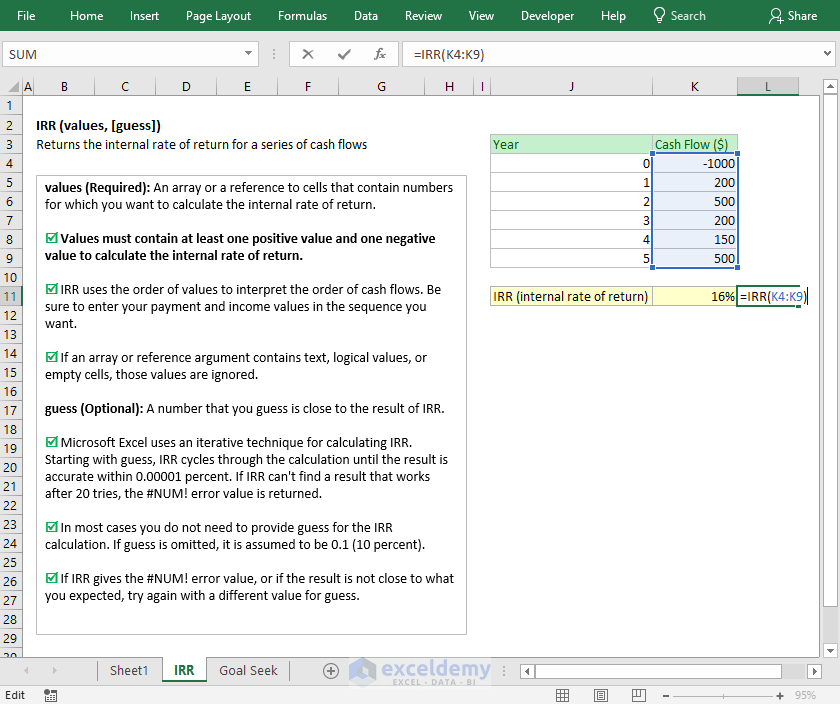And you see the formula returns value 16% (the cell is formatted to percentage).

IRR function is a very simple one to use.

Syntax of IRR function: IRR(values, [guess])

As the values argument, we have passed this cell range K4: K9. Values must contain at least one negative value and one positive value. Otherwise, the IRR function will provide #NUM! error value.

We did not pass any value for the guess argument, it is not necessary actually.

So, this is one way.

## 2) Using IRR function in Excel VBA

IRR function can also be used in Excel VBA.

`Dim IRR_VALUE As Double`

`Static Cash_Flow(6) As Double`

`Cash_Flow(0) = -1000`

`Cash_Flow(1) = 200`

`Cash_Flow(2) = 500`

`Cash_Flow(3) = 200`

`Cash_Flow (4) = 150`

`Cash_Flow (5) = 500`

`IRR_VALUE = Irr(Cash_Flow())`

This code will provide the same IRR value that we have found using IRR Excel function in Excel spreadsheet.

## 3) Calculate internal rate of return using Excel’s Goal Seek Feature

To use Goal Seek feature to find the internal rate of return, you have to understand how to calculate the Net Present Value in Excel.

### What is Net Present Value?

Money that you have now is more valuable than the money that earned later.

If you have \$1000 today, you can invest it in a business, or buy a product today and sell it later, or at least you can deposit it with a bank that gives you interest for your deposits.

Say, you have a trustable bank nearby your location and they give 12% interest for your deposits.

You go to the bank and deposit your \$1000 with the bank on 12% interest rate per year for next 3 years.

Assume that you’re not withdrawing any money from the bank.

After 1 year you will get:

\$1000+ \$1000 x 12%

= \$1000 (1+12%)

= \$1120

So, \$1120 after 1 year is the same as \$1000 today.

After 2 years, you will get:

\$1000 (1+12%) + \$1000(1+12%)(12%)

= \$1000(1+12%)(1+12%)

= \$1000(1+12%)^2

= \$1254.40

So, \$1254.40 after 2 years is the same as \$1000 today.

In the same way, we can conclude that after 3 years, we shall get:

\$1000(1+12%)^3

= \$1404.93

So, \$1404.93 after 3 years is the same as \$1000 today.

We can conclude an equation from the above discussion:

Future value of the money, FV = PV((1+r)^n)

Here,

PV = Present value

r = Interest rate/year

n = Number of years

Reversely, we can calculate the present value of the money with this equation:

PV = FV/((1+r)^n)

We shall use this formula to find the internal rate of return on future cash flows using Excel’s Goal Seek feature.

### But before that, you have to know what is actually the internal rate of return?

Let’s get back to our previous example.

 Year Cashflow (\$) 0 -1000 (your investment) 1 200 2 500 3 200 4 150 5 500

For this set of cash flows, we got the internal rate of return 16% (using Excel’s IRR function). The value is actually 15.715%

If you use this Internal rate of return to calculate the present values of your future cash flows, your initial investment will be balanced out, I mean

-\$1000 + sum of future cash flows = 0

=> sum of future cash flows = initial investment

So, IRR is the interest rate that balances out your initial investment and future cash flows from the investment.

Let’s show you this mathematically.

Here, r = 15.715% = 0.15715

 Year Cashflow Present Values 0 -1000 (your investment) -1000 1 200 200/(1+r)= 172.8385 2 500 500/(1+r)^2= 373.4144 3 200 200/(1+r)^3= 129.0808 4 150 150(1+r)^4= 83.6630 5 500 500/(1+r)^5= 241.003 Total Cashflows = Almost zero (0)

So, I think theoretically you got the whole idea.

### Using Goal Seek feature to find out the internal rate of return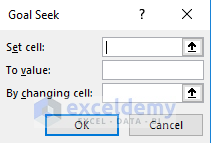Excel’s Goal Seek feature takes three values: Set cell, To value, and By changing cell

You can read it like set cell xxx to value xxx by changing cell xxx.

xxx will be replaced with appropriate values.

Look at the following image. From cells C3 to C7, we have calculated the present values of the future cash flows using this formula: PV = FV/((1+r)^n)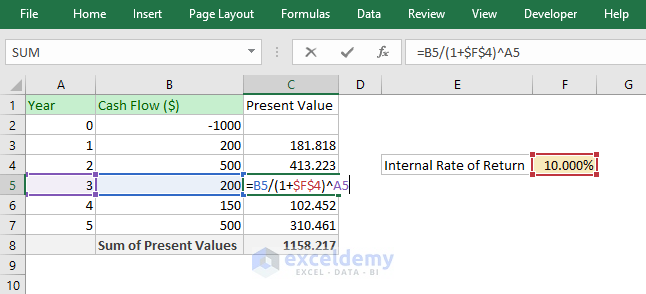I have used an arbitrary value 10% in cell F4. I have used 10%, you can use 5% or any value.

In cell C8, we have summed all those future values.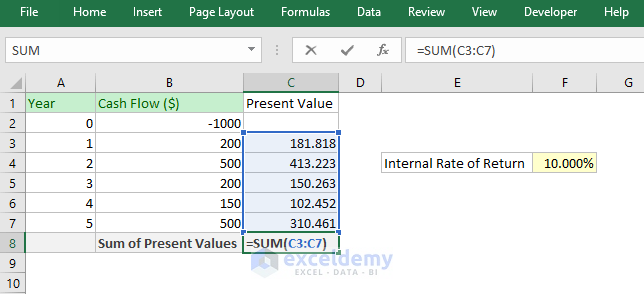Now, we shall use the Excel Goal Seek feature to Set cell C8 (sum of all future cash flows) To value 1000 (equal to initial investment) By changing cell F4 (internal rate of return).

And here is the setting.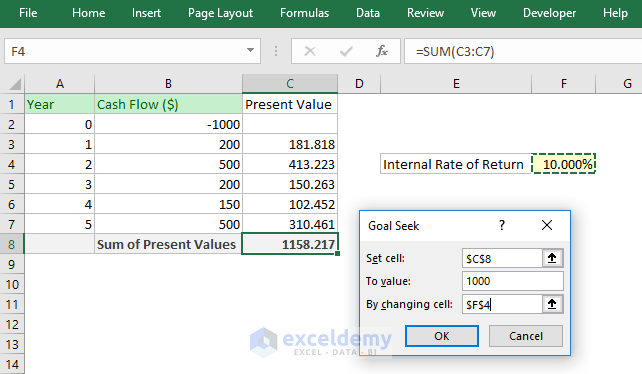And this is what happens when I click on the OK button.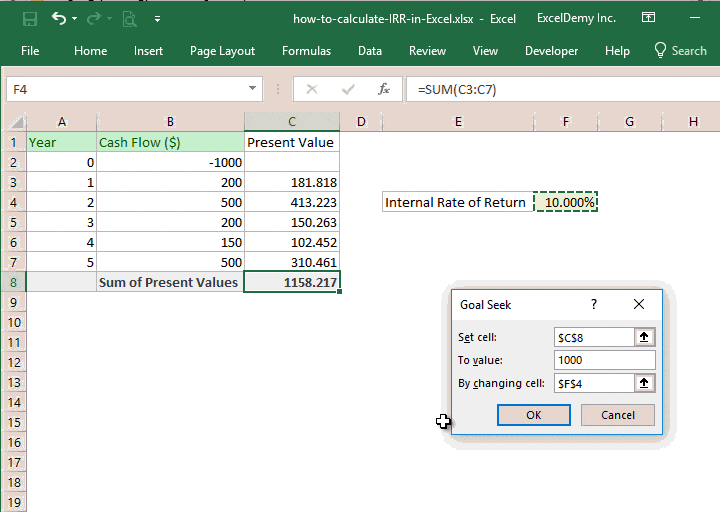Excel Goal Seek feature do the iterations and then comes up with a value that meets all the criteria. It is funny to use.

And we get the same internal rate of return as we got using the Excel’s IRR function.

## 4) How to calculate IRR manually / by hand?

I have shown you two ways of calculating IRR using Excel:

• Using Excel IRR function
• And using Excel’s Goal Seek feature.

Now I will show you how you can calculate IRR manually or by hand.

We shall use the same worksheet to show this method.

In cell range C3 to C7, I have calculated the present values of the cash flows using this formula: PV = FV/((1+r)^n)And in cell C8, we have summed up all the present values of the cash flows.Now, in cell F4, you have to change the value manually to get a nearby value that makes the total present values equal to 1000.

Say, we change the value to 12%. You see the total present value is \$1098.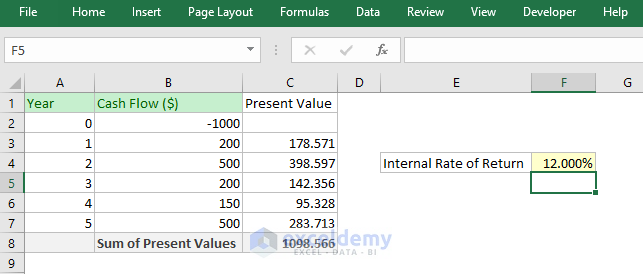Now change the value to say, 16%. You see the value in cell C8 is 993.027.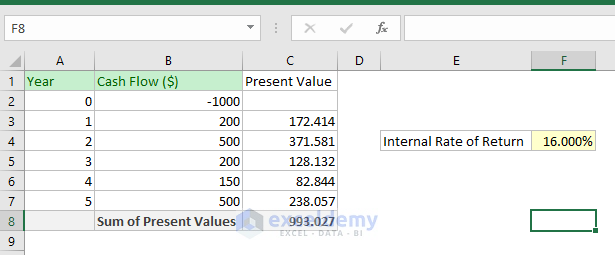This is the manual way. It is not tough but it will take time to get a very close value from this method.

You can also try this method on paper, but that might take even a whole day to find the internal rate of return of some future cash flows.

## 5) How to calculate IRR from NPV (net present value)

When we have shown you how to calculate IRR manually or using by hand in the above section, we have actually used the net present value to calculate IRR.

We have used this formula to find out the present value of future cash flows: PV = FV/((1+r)^n)

Then we have gone through the trial and error method to calculate the IRR.

## 6) How to calculate IRR when cash flows are discrete, not equal periods?

When your cash flows are not regular, you will use Excel’s XIRR function to calculate the internal rate of return.

Say, we have some discrete periods, and the cash flows like the following image. And we want to calculate the IRR from these values.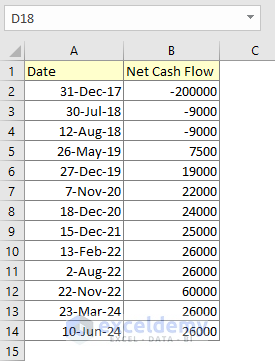In cell B16, we just put this formula: =XIRR(B2:B14,A2:A14).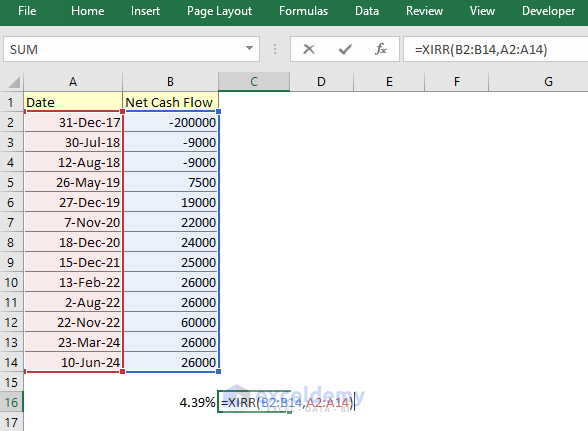Syntax of XIRR Function: XIRR (values, dates, [guess])

XIRR function takes the cash flow values and dates of the cash flows and outputs the internal rate of return (4.39% in our case). I have formatted the cell with %, so you’re seeing the value in percentage.

## 7) How to calculate IRR in Excel for monthly cash flow

In this section, I will show how to calculate IRR in Excel for monthly cash flows.

You are seeing some data in the following image.

Our initial investment is -200000 and it is on 31st Dec 2017.

Then we have shown every month’s cash flow.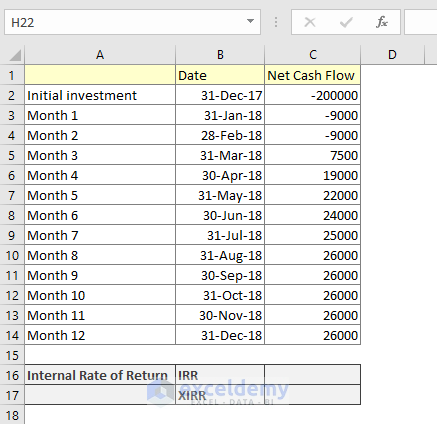IRR function is used to calculate equally payment periods like years. You can use the IRR function to calculate monthly cash flows but as all months are not equal in days, so it is better to use Excel’s XIRR function.

XIRR function is used to calculate the internal rate of return of cash flows that are not periodic. We have seen how to use the XIRR function in the previous section.

To show you the differences between IRR and XIRR, I have calculated both values.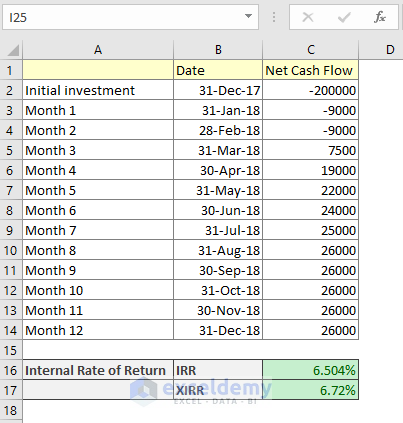You see there is a difference between the values.

Note: When you will use the IRR function to calculate the internal rate of return for monthly cashflows, you will multiply the IRR value by 12 as IRR has calculated the monthly rate of return, not yearly.

## 8) IRR for Uneven cash flows

With the word “Uneven cash flows”, I mean cash flows that are not periodic. In this case, as we discussed in one of our above sections, you will use Excel’s XIRR function.

## 9) How to calculate MIRR in Excel (modified IRR)

Say, you had taken a loan of amount \$5000 at the interest rate of 12% a year to invest in a project. The will give you periodic cash flows like the following image.

What you do is you reinvest the cash flows that come from this existing project in a new project. The new project’s possible internal rate of return is, say, 20%.

So, what is your actual (or modified) internal rate of return for this existing project?

In this situation, you can use Excel’s MIRR function.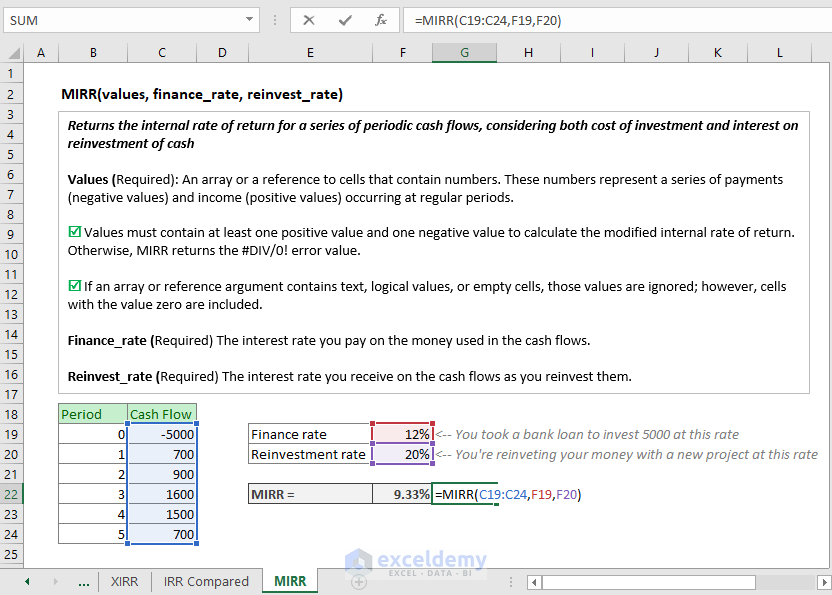In cell F22, we have used Excel’s MIRR function and the function returns a 9.33% modified internal rate of return for this project.

Microsoft Excel’s MIRR function returns the modified internal rate of return for a set of cash flows.

Syntax of Excel MIRR function: MIRR (values, finance_rate, reinvest_rate)

All the arguments are required.

## What is a good IRR?

It is a very tough question for answering. Normally IRR value is compared with a fixed value like Bank Interest Rate, or Government Bond rate as you can earn these incomes idly without doing anything and risking anything.

And IRR is also compared the project to project basis.

In the following image, you’re seeing data of two projects and their expected revenue for over the next 5 years.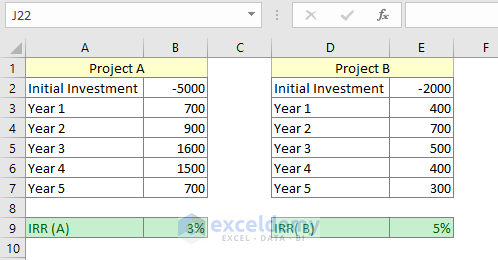After calculating the IRR of these projects, it seems that Project B would be more potential for the company.

## Conclusion

So, these are my ways of calculating IRR using Excel, the world’s most popular spreadsheet program. If you have any feedback or corrections with this article, please let me know in the comment section or email me using my email ID on the contact page.

Do you know another way of calculating IRR using Excel? I am eager to know.

Hello!
Welcome to my Excel blog! It took me some time to be a fan of Excel. But now I am a die-hard fan of MS Excel. I learn new ways of doing things with Excel and share here. Not only how to guide on Excel, but you will get also topics on Finance, Statistics, Data Analysis, and BI. Stay tuned!

1. ReplyClovis Miglioli Mar 15, 2019 at 7:22 AM

Kawser
Very understandable and effective article regarding Internal Rate of Return ( IRR). I have one point to complain on comparison IRR and XIRR. It seems that as IRR is a monthly rate and to compute it annually we must use a compound form. as (IRR ^12) and not multiplied. Doing so we have IRR = 0,542% monthly – and ( 1,00542^12) we get 6,70 % – quite the same om XIRR ( 6.72%).

2. Reply